# String Matrix

String Matrix: In this article, we are going to see how backtracking can be used to solve following problems?
Submitted by Radib Kar, on February 13, 2020

Description:

In this article, we are going to see how backtracking can be used to solve following problems?

Problem statement:

A matrix of characters schematically represents a swamp. The swamp is composed of muddy areas, represented with the '.' character, and rocky areas, represented with the '*' character.

Example of swamp:

```    **.......
.......**.
...........
......**..
..........
```

Write a C program that searches a path in the swamp, from the left to the right, without jumps, only including consecutive rocky areas. Suppose that each rocky area can have at most one other rocky area on its right (there are no branches), i.e., either on the same row, or in the previous row, or the following one. The program shall print the row sequence of the path (the columns are implicit – there shall be a rocky area for each column), or report that no path exists.

Explanation with example:

Let's discuss the following input:

```    **.*.*....*
..*.*...**
*...*.*....
.*.*.*.*.*.
..*.*...*.*
```

Let's display the input in a 2D matrix for better visualization.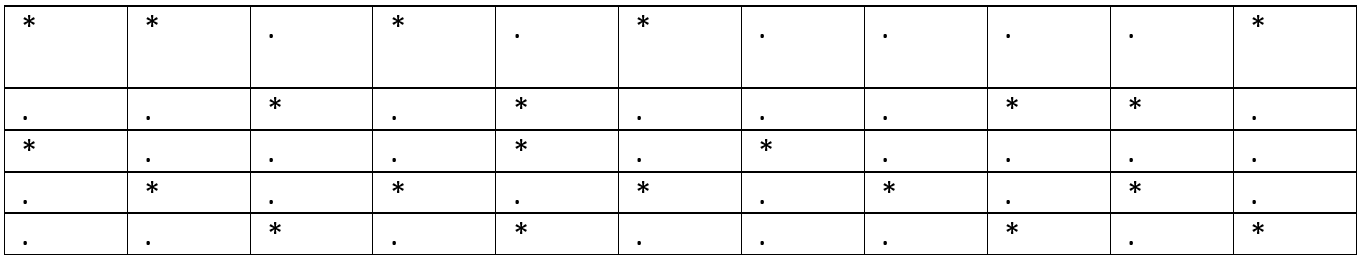Let's start from the 0th column (0 indexing),

There is a rock in the row 0

Start from (0, 0)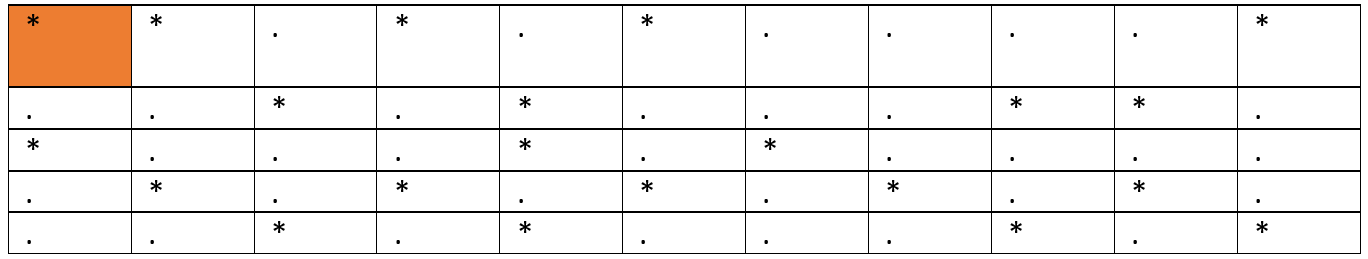Next rock that can be reached without any jump (0, 1)

Path: (0, 0) -> (0, 1)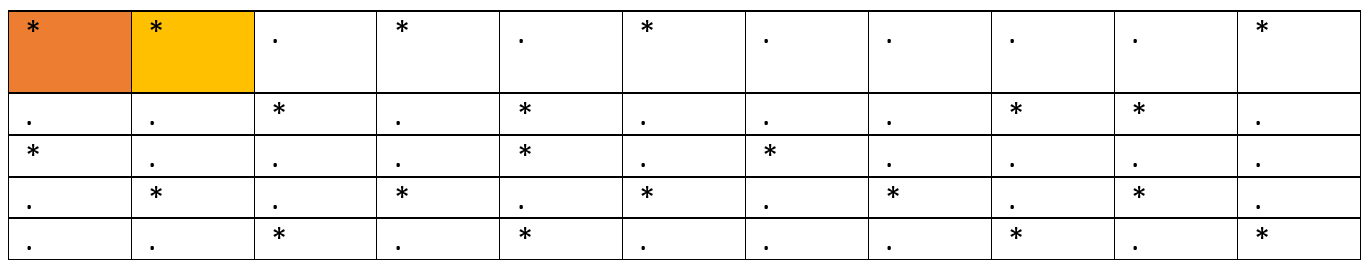Next rock that can be reached without any jump (1, 2)

Path: (0, 0) -> (0, 1) -> (1, 2)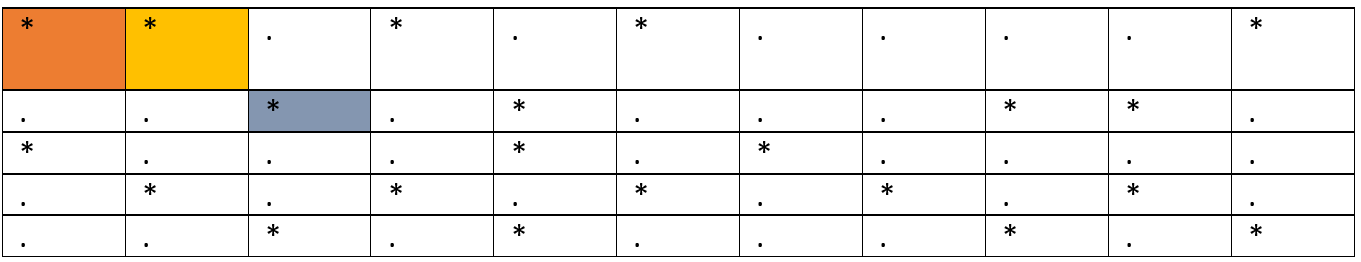Next rock that can be reached without any jump (0, 3)

Path: (0, 0) -> (0, 1) -> (1, 2)-> (0, 3)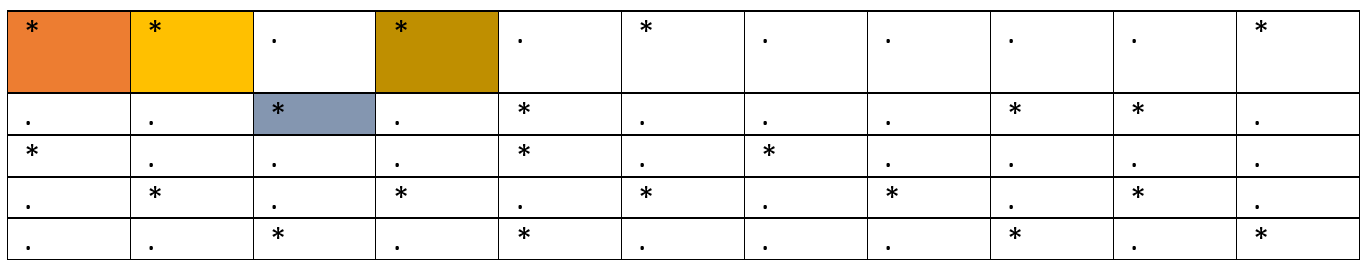Next rock that can be reached without any jump (1, 4)

Path: (0, 0) -> (0, 1) -> (1, 2)-> (0, 3) -> (1, 4)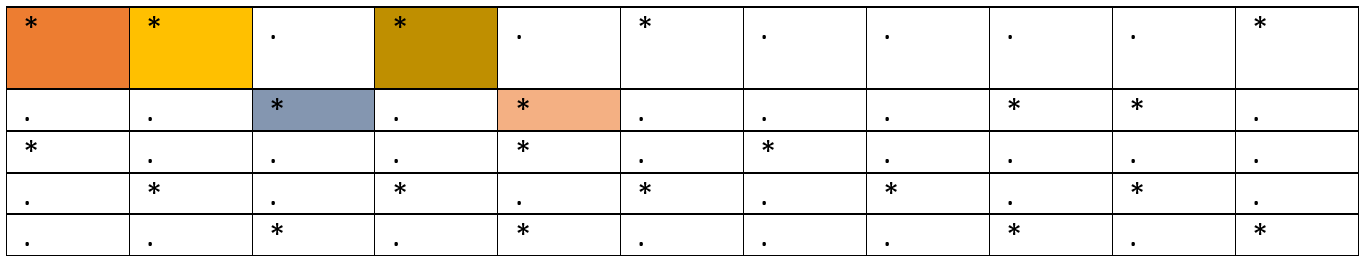Next rock that can be reached without any jump (0, 5)

Path: (0, 0) -> (0, 1) -> (1, 2)-> (0, 3) -> (1, 4) -> (0, 5)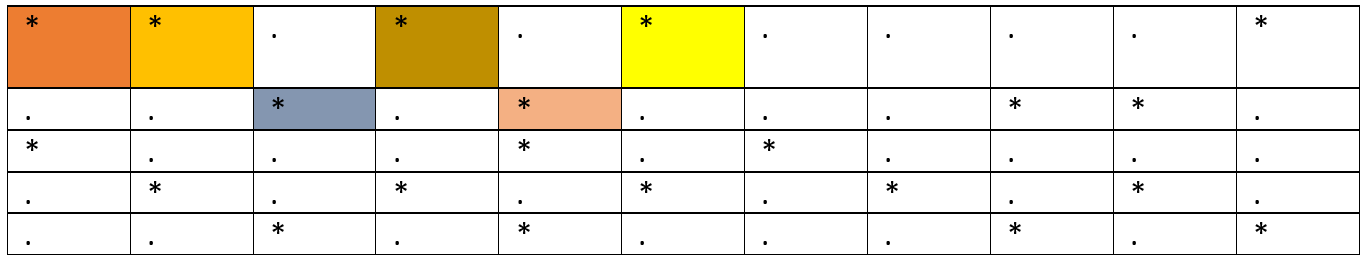Now, there is no next rock that can be reached from here, so we need to backtrack and find other alternatives. (red filled area refers that already explored but failed).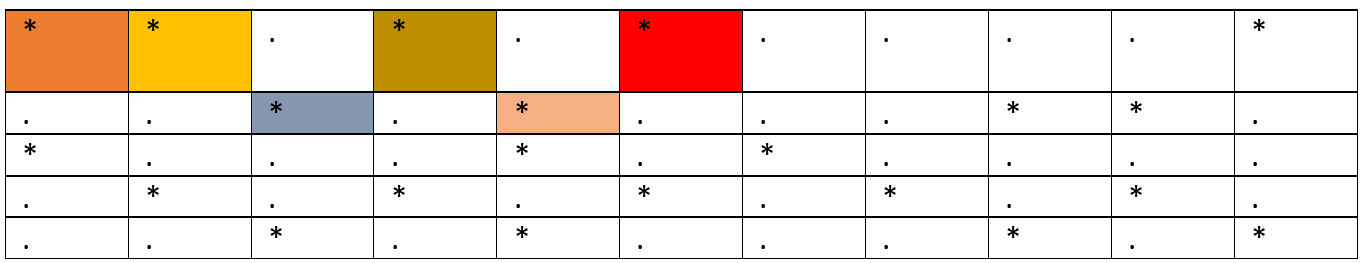So, we backtrack to previous state and the last point on our path is (1, 4). (0, 5) is already explored and failed option. Looking for alternative there is no more rock that can be reached from here. We need to backtrack again.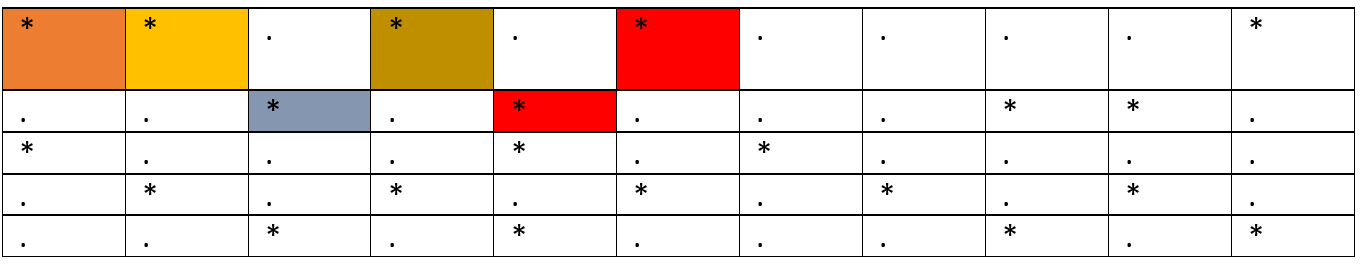Such backtrack will ultimately yield the following state.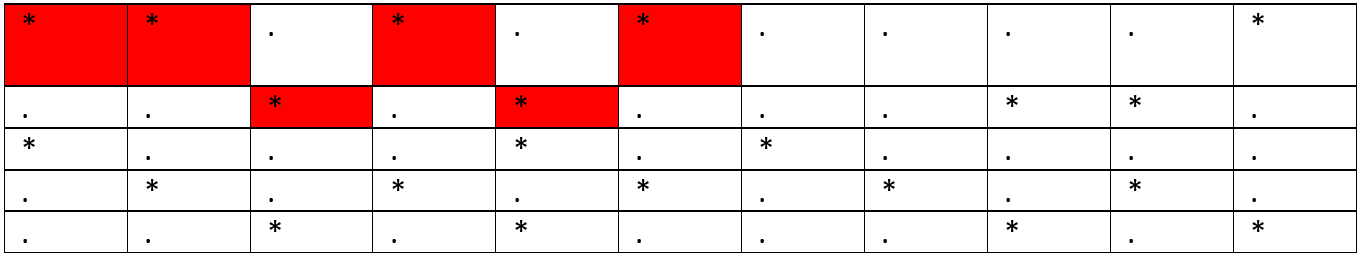So basically, all the path we had explored is failed.

We will start fresh from (2, 0) and start the same procedure again. If you keep doing you can see that the ultimate result is: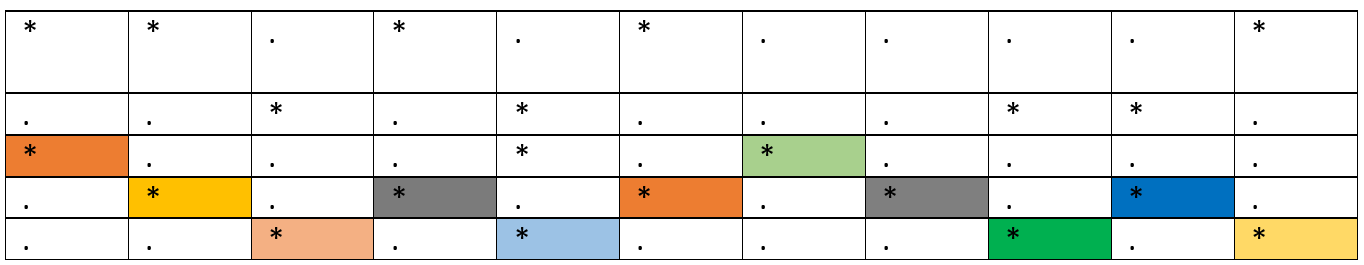This is what backtrack is, explore through all the choices possible, backtrack if there is no next move. Of course, this kind of search technique is greedy, but it helps sometimes when you have no choices.

N.B: there can be multiple paths possible, depends on your implementation. If you terminate the program while the goal is reached it will return one path only. But if you keep exploring other possibilities as well, you can find other possible paths.

Algorithm:

```    1.	Start: start from initial point
2.	Explore one from the possible next moves
3.	If no more moves possible & goal is not reached
backtrack and choose one from other alternatives.
4.	If goal is reached, success
5.	Else failure.
```

C Implementation:

```#include <stdio.h>
#define ROW 25
#define COL 80

char arr[ROW][COL];
int vis[COL],store[COL];

int issafe(int vis[],int curr,int curc,int r,int c){
//printf("%c\n",arr[curr][curc]);
if(curr<0 || curr>=r || curc<0 || curc>=c || arr[curr][curc]=='.')
return 0;

return 1;
}

int findpath(int vis[],int store[],int curr,int curc,int r,int c){
//base case
if(curc==c){
//store[curc]=curr;
printf("The path can be: ");

for(int i=0;i<c;i++){
printf("%d ",store[i]);
}
printf("\n");
return 1;
}

if(issafe(vis,curr,curc,r,c)){
vis[curc]=1;
store[curc]=curr;
//printf("%d\n",store[curc]);
if(findpath(vis,store,curr,curc+1,r,c))
return 1;
if(findpath(vis,store,curr+1,curc+1,r,c))
return 1;
if(findpath(vis,store,curr-1,curc+1,r,c))
return 1;

vis[curc]=0;
store[curc]=0;
return 0;
}
else{
return 0;
}
}

int main()
{
// FILE *fptr;
// fptr = fopen("input.txt", "r");
// if (fptr == NULL)
// {
//     printf("Cannot open file \n");
//     exit(0);
// }

int r,c;

printf("Enter number of rows and column\n");
scanf("%d %d",&r,&c);

printf("Enter the string matrix\n");
for(int i=0;i<r;i++){
scanf(" %[^\n]",arr[i]);
}

// for(int i=0;i<r;i++){
//     for(int j=0;j<c;j++){
//         printf("%c ",arr[i][j]);
//     }
//     printf("\n");
// }

int flag=0;
for(int i=0;i<r;i++){
for(int j=0;j<c;j++)
vis[j]=0;
for(int j=0;j<c;j++)
store[j]=0;
if(findpath(vis,store,i,0,r,c)){
flag=1;
//don't break here, if you need all possible paths
break;
}
}

if(flag==0)
printf("No path there\n");

return 0;
}
```

Output

```Enter number of rows and column
5 11
Enter the string matrix
**.*.*....*
..*.*...**.
*...*.*....
.*.*.*.*.*.
..*.*...*.*
The path can be: 2 3 4 3 4 3 2 3 4 3 4
```

What's New (MCQs)

Top Interview Coding Problems/Challenges!

Languages: » C » C++ » C++ STL » Java » Data Structure » C#.Net » Android » Kotlin » SQL
Web Technologies: » PHP » Python » JavaScript » CSS » Ajax » Node.js » Web programming/HTML
Solved programs: » C » C++ » DS » Java » C#
Aptitude que. & ans.: » C » C++ » Java » DBMS
Interview que. & ans.: » C » Embedded C » Java » SEO » HR
CS Subjects: » CS Basics » O.S. » Networks » DBMS » Embedded Systems » Cloud Computing
» Machine learning » CS Organizations » Linux » DOS
More: » Articles » Puzzles » News/Updates

© https://www.includehelp.com some rights reserved.